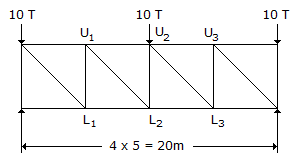# Civil Engineering - Strength of Materials

### Exercise :: Strength of Materials - Section 1

26.

The maximum twisting moment a shaft can resist, is the product of the permissible shear stress and

 A. moment of inertia B. polar moment of inertia C. polar modulus D. modulus of rigidly.

Explanation:

No answer description available for this question. Let us discuss.

27.

For a simply supported beam with a central load, the bending moment is

 A. least at the centre B. least at the supports C. maximum at the supports D. maximum at the centre.

Explanation:

No answer description available for this question. Let us discuss.

28.

For a given material Young's modulus is 200 GN/m2 and modulus of rigidity is 80 GN/m2. The value of Poisson's ratio is

 A. 0.15 B. 0.2 C. 0.25 D. 0.3 E. 0.4

Explanation:

No answer description available for this question. Let us discuss.

29.

The ratio of the moment of inertia of a circular plate and that of a square plate for equal depth, is

 A. less than one B. equal to one C. more than one D. equal to 3π/16 E. none of these.

Explanation:

For circular plate, MI = π*d^4/64.

For square plate, MI = d^4/12.

So, Ratio = MI of circular plate/MI of square plate.

= 3*π/16.

30.

The force in member U2L2 of the truss shown in below figure, isA. 10 T tension B. 10 T compression C. zero D. 15 T compression.